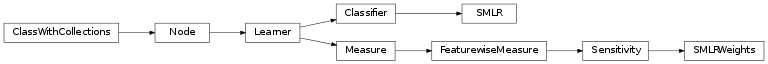# mvpa2.clfs.smlr¶

Sparse Multinomial Logistic Regression classifier.Functions

Classes

 `SMLR`(**kwargs) Sparse Multinomial Logistic Regression `Classifier`. `SMLRWeights`(clf[, force_train]) `SensitivityAnalyzer` that reports the weights SMLR trained

Exceptions

 `SMLR`(**kwargs) Sparse Multinomial Logistic Regression `Classifier`. `SMLRWeights`(clf[, force_train]) `SensitivityAnalyzer` that reports the weights SMLR trained